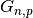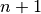Functions¶

netconfig package¶

buffers module¶

Function to assign and manipulate buffer sizes of network interfaces.

 clear_buffer_sizes(topology) Remove all buffer sizes from the topology. get_buffer_sizes(topology) Returns all the buffer sizes. set_buffer_sizes_bw_delay_prod(topology[, …]) Assign a buffer sizes proportionally to the product of link bandwidth and average network RTT. set_buffer_sizes_constant(topology, buffer_size) Assign a constant buffer size to all selected interfaces set_buffer_sizes_link_bandwidth(topology[, …]) Assign a buffer sizes proportionally to the bandwidth of the interface on which the flush.

capacities module¶

Functions to assign and manipulate link capacities of a topology.

Link capacities can be assigned either deterministically or randomly, according to various models.

delays module¶

Functions to assign and manipulate link delays.

 clear_delays(topology) Remove all delays from the topology. get_delays(topology) Returns all the delays. set_delays_constant(topology[, delay, …]) Assign a constant delay to all selected links set_delays_geo_distance(topology, specific_delay) Assign a delay to all selected links equal to the product of link length and specific delay.

nodeconfig module¶

Functions to deploy and configure protocol stacks and applications on network nodes

 add_application(topology, node, name[, …]) Add an application to a node add_stack(topology, node, name[, properties]) Set stack on a node. clear_applications(topology) Remove all applications from all nodes of the topology clear_stacks(topology) Remove all stacks from all nodes of the topology get_application_names(topology, node) Return a list of names of applications deployed on a node get_application_properties(topology, node, name) Return a dictionary containing all the properties of an application deployed on a node get_stack(topology, node[, data]) Return the stack of a node, if any remove_application(topology, node[, name]) Remove an application from a node remove_stack(topology, node) Remove stack from a node

weights module¶

Functions to assign and manipulate link weights to a network topology.

 clear_weights(topology) Remove all weights from the topology. get_weights(topology) Returns all the weights. set_weights_constant(topology[, weight, links]) Assign a constant weight to all selected links set_weights_delays(topology) Assign link weights to links proportionally their delay. set_weights_inverse_capacity(topology) Assign link weights to links proportionally to the inverse of their capacity.

traffic package¶

eventscheduling module¶

Functions and classes for creating and manipulating event schedules.

An event schedule is simply a list of events each labelled with a time and a number of properties.

An event schedule can be read and written from/to an XML files with provided functions.

 deterministic_process_event_schedule(…) Return a schedule of events separated by a fixed time interval poisson_process_event_schedule(avg_interval, …) Return a schedule of Poisson-distributed events read_event_schedule(path) Read event schedule from an XML file write_event_schedule(event_schedule, path[, …]) Write an event schedule object to an XML file.

trafficmatrices module¶

Functions and classes for creating and manipulating traffic matrices.

The functions of this class allow users to synthetically generate traffic matrices with given statistical properties according to models proposed in literature.

The output of this generation is either a TrafficMatrix or a TrafficMatrixSequence object.

A traffic matrix or a sequence of matrices can be read and written from/to an XML files with provided functions.

 link_loads(topology, traffic_matrix[, …]) Calculate link utilization given a traffic matrix. read_traffic_matrix(path[, encoding]) Parses a traffic matrix from a traffic matrix XML file. sin_cyclostationary_traffic_matrix(topology, …) Return a cyclostationary sequence of traffic matrices, where traffic volumes evolve over time as sin waves. static_traffic_matrix(topology, mean, stddev) Return a TrafficMatrix object, i.e. stationary_traffic_matrix(topology, mean, …) Return a stationary sequence of traffic matrices. validate_traffic_matrix(topology, traffic_matrix) Validate whether a given traffic matrix and given topology are compatible. write_traffic_matrix(traffic_matrix, path[, …]) Write a TrafficMatrix or a TrafficMatrixSequence object to an XML file.

topologies package¶

datacenter module¶

Functions to generate commonly adopted datacenter topologies.

Each topology generation function returns an instance of DatacenterTopology

 bcube_topology(n, k) Return a Bcube datacenter topology, as described in [R48460de4c968-1]: fat_tree_topology(k) Return a fat tree datacenter topology, as described in [Rdaad0f90b4be-1] three_tier_topology(n_core, n_aggregation, …) Return a three-tier data center topology. two_tier_topology(n_core, n_edge, n_hosts) Return a two-tier datacenter topology.

parsers module¶

Functions to parse topologies from datasets or from other generators.

 parse_abilene(topology_path[, links_path]) Parse the Abilene topology. parse_ashiip(path) Parse a topology from an output file generated by the aShiip topology generator parse_brite(path[, capacity_unit, …]) Parse a topology from an output file generated by the BRITE topology generator parse_caida_as_relationships(path) Parse a topology from the CAIDA AS relationships dataset parse_inet(path) Parse a topology from an output file generated by the Inet topology generator parse_rocketfuel_isp_map(path) Parse a network topology from RocketFuel ISP map file. parse_rocketfuel_isp_latency(latencies_path) Parse a network topology from RocketFuel ISP topology file (latency.intra) with inferred link latencies and optionally annotate the topology with inferred weights (weights.infra). parse_topology_zoo(path) Parse a topology from the Topology Zoo dataset.

randmodels module¶

Functions to generate random topologies according to a number of models.

The generated topologies are either Topology or DirectedTopology objects.

 barabasi_albert_topology(n, m, m0[, seed]) Return a random topology using Barabasi-Albert preferential attachment model. erdos_renyi_topology(n, p[, seed, fast]) Return a random graph(Erdos-Renyi graph, binomial graph). extended_barabasi_albert_topology(n, m, m0, p, q) Return a random topology using the extended Barabasi-Albert preferential attachment model. glp_topology(n, m, m0, p, beta[, seed]) Return a random topology using the Generalized Linear Preference (GLP) preferential attachment model. waxman_1_topology(n[, alpha, beta, L, …]) Return a Waxman-1 random topology. waxman_2_topology(n[, alpha, beta, domain, …]) Return a Waxman-2 random topology.

simplemodels module¶

Generate canonical deterministic topologies

 chord_topology(m[, r]) Return a Chord topology, as described in [R409142fb3296-1]: dumbbell_topology(m1, m2) Return a dumbbell topology consisting of two star topologies connected by a path. full_mesh_topology(n) Return a fully connected mesh topology of n nodes k_ary_tree_topology(k, h) Return a balanced k-ary tree topology of with depth h line_topology(n) Return a line topology of n nodes ring_topology(n) Return a ring topology of n nodes star_topology(n) Return a star (a.k.a hub-and-spoke) topology ofnodes

topology module¶

Basic functions and classes for operating on network topologies.

 fan_in_out_capacities(topology) Calculate fan-in and fan-out capacities for all nodes of the topology. od_pairs_from_topology(topology) Calculate all possible origin-destination pairs of the topology. rename_edge_attribute(topology, old_attr, …) Rename all edges attributes with a specific name to a new name rename_node_attribute(topology, old_attr, …) Rename all nodes attributes with a specific name to a new name read_topology(path[, encoding]) Read a topology from an XML file and returns either a Topology or a DirectedTopology object write_topology(topology, path[, encoding, …]) Write a topology object on an XML file

autonetkit module¶

This module contains function for converting FNSS Topology objects into NetworkX graph objects compatible with AutoNetKit and viceversa.

 from_autonetkit(topology) Convert an AutoNetKit graph into an FNSS Topology object. to_autonetkit(topology) Convert an FNSS topology into a NetworkX graph object compatible for AutoNetKit.

jfed module¶

Provides function to convert an FNSS Topology object into a jFed rspec file and viceversa.

jFed <http://jfed.iminds.be/>_ is a Java-based framework to support the integration of federated testbed, developed by iMinds <http://www.iminds.be/>_ in the contex of the Fed4FIRE <http://www.fed4fire.eu/>_ project funded by the Framework Programme 7 (FP7) of the European Union.

 from_jfed(path) Read a jFed RSPEC file and returns an FNSS topology modelling the network topology of the jFed experiment specification. to_jfed(topology, path[, testbed, encoding, …]) Convert a topology object into an RSPEC file for jFed

mn module¶

This module contains function to convert FNSS topologies into Mininet topologies and viceversa.

 from_mininet(topology) Convert a Mininet topology to an FNSS one. to_mininet(topology[, switches, hosts, …]) Convert an FNSS topology to Mininet Topo object that can be used to deploy a Mininet network.